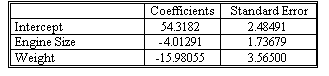### Why should I choose AnalystNotes?

Simply put: AnalystNotes offers the best value and the best product available to help you pass your exams.

##### Subject 3. Confidence Intervals for Regression Coefficients in a Multiple Regression
A 100 (1 - α)% confidence interval for a coefficient βk takes the form
(bk - tα/2, v sbk, bk + tα/2, v sbk)

where sbk is the estimated standard deviation of bk, and tα/2, v is the critical value of the t distribution that has v = (T - K - 1) degrees of freedom such that P(t > tα/2, v) = α/2.

Let's construct 95% confidence intervals for each of the regression coefficients for the sample regression equation in los a.We have T = 10 observations and K = 2 explanatory variables in the model, so the appropriate degrees of freedom is v = 10 - 2 - 1 = 7. To construct a 95% confidence interval, we need the critical values from the t distribution having 7 degrees of freedom. We obtain t0.025, 7 = 2.365.

The desired confidence intervals are:

β0: 54.318 ± 2.365(2.485) or (48.441, 60.195)
β1: -4.013 ± 2.365(1.737) or (-8.121, 0.095)
β2: -15.981 ± 2.365(3.565) or (-24.412, -7.55)

Recall that the variable X1 is engine size, so the coefficient b1 measures the estimated influence of engine size on gasoline mileage. We would expect this influence to be negative; that is, the larger the engine, the lower the gasoline mileage. However, observe that the 95% confidence interval for β1 contains the value 0. This implies that if we performed a two sided test using α = 0.05, we would not reject H0: β1 = 0.

The confidence interval for β2 is fairly wide, which implies that our sample of data has not provided a very precise estimate of the effect of car weight on gas mileage. Nevertheless, the confidence interval for β2 does not contain the value 0, so we can feel quite confident that increasing a car's weight does have a negative effect on gas mileage.

Learning Outcome Statements

e. calculate and interpret a predicted value for the dependent variable, given an estimated regression model and assumed values for the independent variables;

CFA® 2023 Level I Curriculum, Volume 1, Module 2